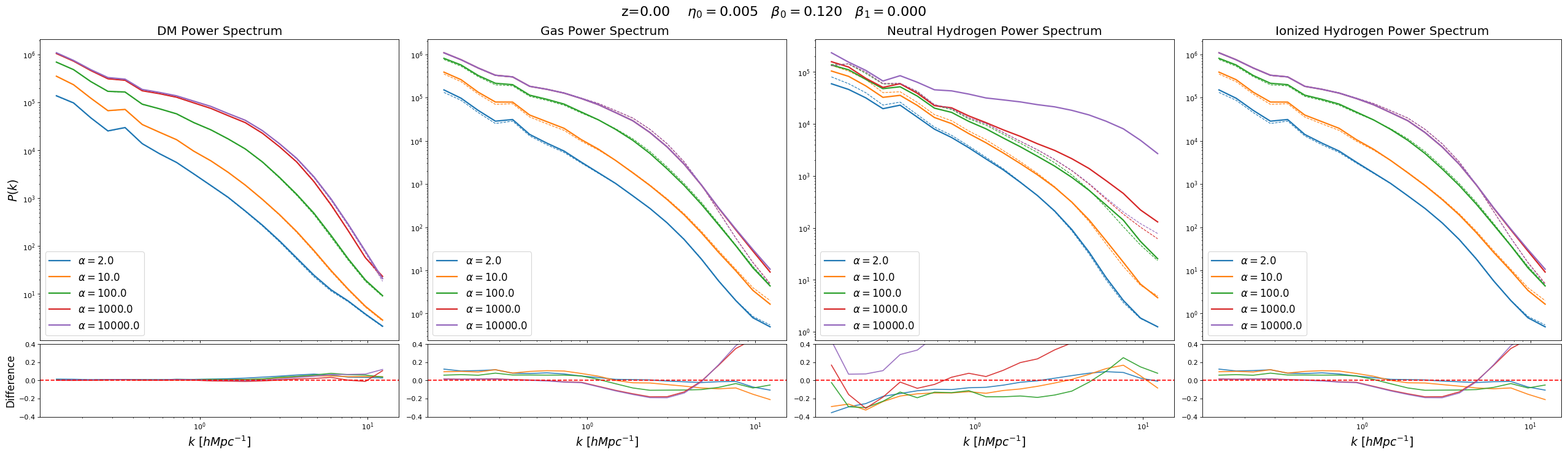Comparison of the power spectrum when limiting the overdensities on both simulations according to the following rule:

Dual Energy Parameters: $\eta=0.005$ $\beta_0 = 0.12$ $\beta_1 = 0.0$

Redshift = 0